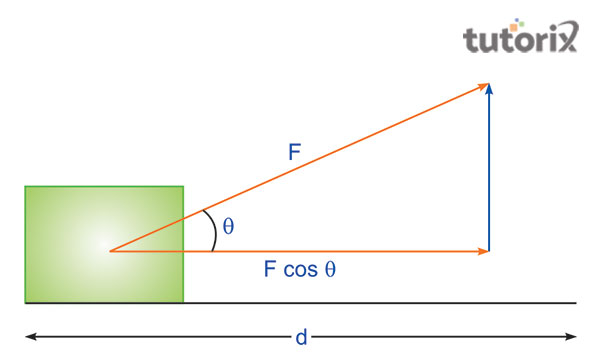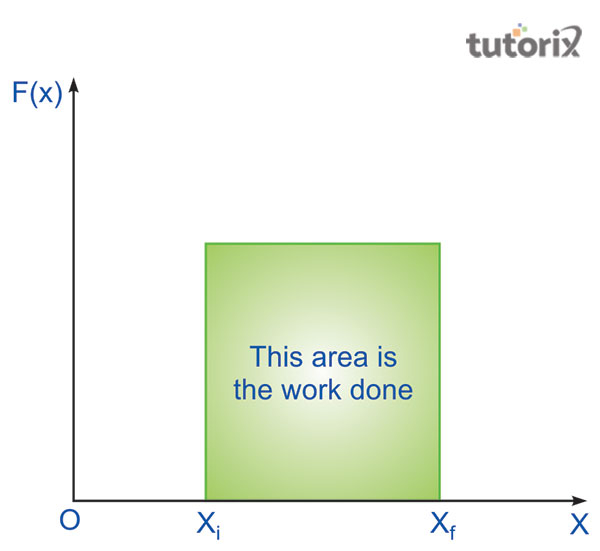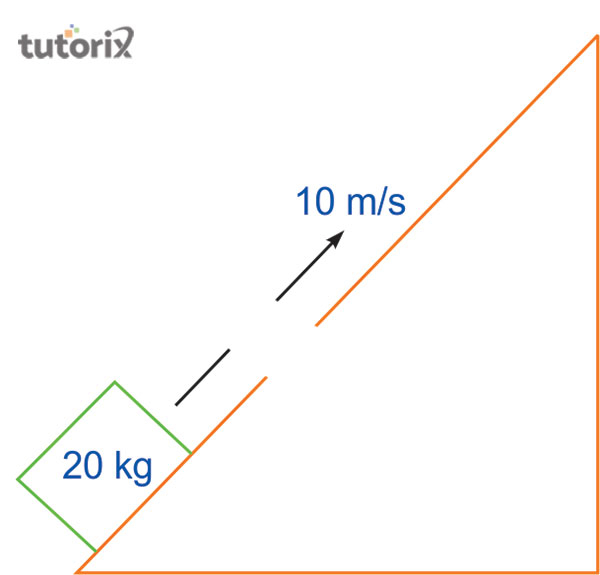# Derivation of Work Energy Theorem

## Introduction

The Work-Energy Theorem outlines that the net completed work by the forces that are applied to the machinery body from external sources that is equivalent to the alternation rate of the kinetic energy of a particular object. The Work-Energy Theorem on an object is done by all of the forces rather than applied to a single force. According to the Work-Energy Theorem, the kinetic energy is mainly defined as the required work power for accelerating the object from the resting phase to a kind of state that required velocity.

## What is the work-energy theorem?Figure 1: Diagram of work-energy theorem

The equation of the work-energy theorem is presented by the formula W= FdcosӨ where W refers to work, F stands for the external force that is applied to an object for the displacement from the previous position, and d denotes the distance between to different position of an object. The virtue of the motion of an object caused by the energy possessed is referred to as the kinetic energy.

## Derivation of the work-energy theorem

As per the equation of motion the derivation of the work-energy theorem can be defined as v2 = u2 + 2as where V refers to the ultimate velocity of an object, u stands for the preliminary velocity of an object, a stands for constant acceleration and s denotes the object displacement.

As per the second law of Newton F = ma, therefore the equation can also be written as 1/2 mv2 - 1/2 mu2 = F.d

Here, W is equivalent to F.d as well as K.E. s equivalent to (mv2)/2 then the equation can be presented as Kf – Ki = W.

Therefore, ΔK = W in which ΔK stands for Kf – Ki that is the alternation of the kinetic energy.

So, it can be said that work done on a specific object is equivalent to the alternation of the kinetic energy.

## Work-energy theorem for the variable force

The external force that commonly comes across in daily life is referred to as the variable force. From the perspective of the derivation of the work-energy theorem the formula of the variable force is hectic.

It can be presented as $\Delta W = F\left ( x \right ) \Delta x$ where different of work has relied on the applied external force and the difference of x.Figure 2: Work-energy theorem for the variable force

With the change of kinetic force the formula is described as dK/dt = d/dt(1/2 mv2) and as per the second law of Newton the acceleration is changed to dK/dt = mav.

As F = ma then the rate of velocity is changed over the time, so the equation is changed to dK/dt = F.dx/dt and in case of the derivation of time-released from both sides then the equation represents as the dK = Fdx or ΔK = W.

Since the equation proved that, the work-energy theorem relies on the variable force.

## Work-energy theorem for constant force derivation

According to the second law of Newton, F = ma where m refers to the mass of the object that moves with the influence of the constant force F and the a stands for the object acceleration.

For the constant force derivation the equation is Fd = 1/2 m.v22 – 1/2 m v12 where F refers to the constant force, and mv stands for the mass velocity of an object. In the formula, d refers to the distance between the initial position as well as the final position of the object and here the equation stands for K2 = m.v12/2, which stands for the ultimate kinetic energy of a specific object.Figure 3: Work-energy theorem for constant force derivation

The quantity of the object is K1=mv12/2 which is the preliminary kinetic energy of a specific object.

Then the equation comes up with W = K2-K1=ΔK in which ΔK stands for the alternation of kinetic energy of that specific object.

From this particular equation, it can be stated that work done by the force of a specific object is equivalent to the alternation of the kinetic energy of that object.

## Features of the work-energy theorem and its application

• During the constant speed of an object, kinetic energy is not changed and the work done by resultant force is equivalent to zero.

• Energy is manifested after the work is completed so the energy can be decreased with the allowance of the object that is undertaken by the other particles.

• It is very useful to understand the situation of the movement of a rigid body by the numerous forces.

## Interesting facts on the work-energy theorem

• It states that the work done by the external forces on an object is equivalent to the kinetic energy of that particular object.

• The second law of Newton is very much applicable in the work-energy theorem.

• Work on an object can be positive as well as negative.

## Conclusion

Work refers to the force that is applied to the object by the external force for the displacement from the previous position. It is equivalent to the component product, the direction force for displacement along with the displacement magnitude of a specific subject. Work can be measured by Joule, which is its SI unit. Work done denotes the total energy that is transferred from a single object with the application of external force that affects the displacement direction of an object.

## FAQs

Q.1 What does the work-energy theorem prove?

Ans. The work-energy theorem states the relationship between kinetic energy and work done on an object. Work is said to be done when applied kinetic force on the object results in the displacement of the object.

Q.2 What is the reason for the work-energy theorem is not applicable to all types of forces?

Ans. All types of forces are not comprised of non-conservative factors so the work-energy theorem is not applicable to all types of forces. The equation of the work-energy theorem is W = KEf - KEi = 1/2mvf2 - 1/2mvi2 .

Q.3 Why the work is sometimes negative?

Ans. When the force is applied in the opposite direction then the work is negative. In case of force is applied in the same direction the work will be positive.

Q.4 What is the significance of the work-energy theorem?

Ans. The work-energy theorem is very helpful for the measurement of the kinetic energy of an object. It is also applicable for the measurement of force.